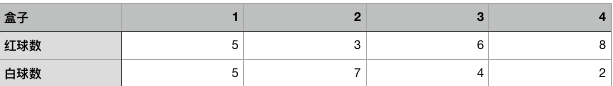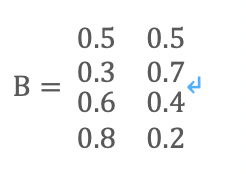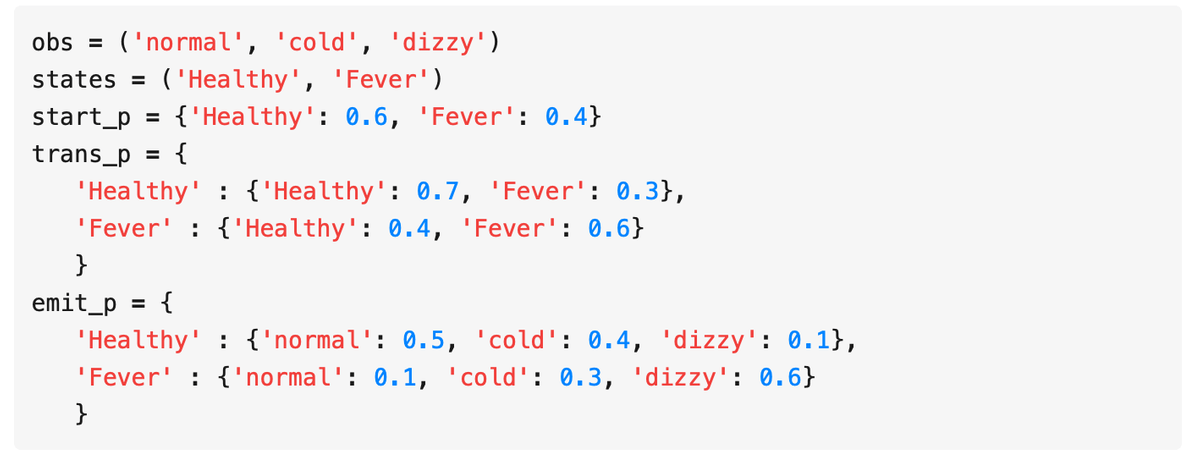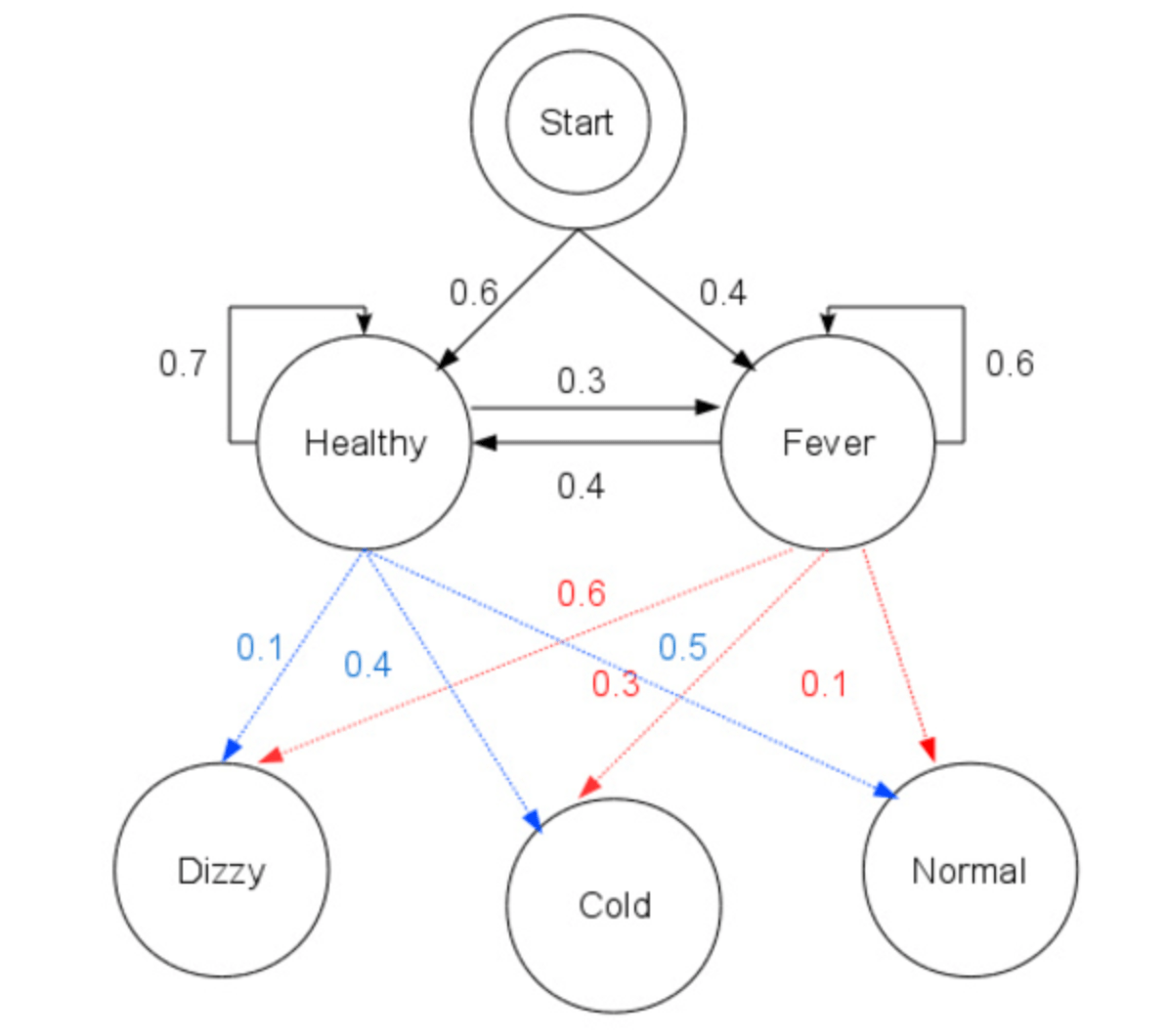# 隐马尔可夫模型HMM

## 「统计学习」系列

· 统计学习

HMM是可用于标注问题的统计学习模型,描述由潜在的马尔可夫链(Markov chain)随机生成预测序列的过程 ── 属于生成模型。

HMM在语音识别、NLP、生物信息表达(特别,基因研究)、模式识别等领域有着广泛应用。

HMM由几个核心组成要求, 第一部分是序列信息:

• 状态序列:隐藏的Markov chain随机生成的状态序列;    state sequence
• 观测序列:每个状态会生成一个观测,由此产生一连串的观测记录, 叫做观测序列;  observation sequence
• 位置标号:序列的每一个位置可以视作为一个时刻, 可以是等间距的时间序列, 也可以相隔不同分/时/秒的序列。

• 初始概率分布
• 状态转移概率分布
• 观测概率分布

Q 是所有可能的状态集合, V 是所有可能的观测的集合:

Q = {q1,q2, ...qN}

V = {v1,v2, ...vM}

N 是状态集的状态数, M是所有可能的观测数。

I = (i1, i2, ... , iT)

O = (o1, o2, ... , oT)

A是状态转移概率矩阵:

A = [aij] N*N

B是观测概率矩阵:

B = [bj(k)] N*M

bj(k)表示 状态在qj下生成观测的vk概率, k = 1,2, ... M, j = 1,2, ..., N

π 表示初始状态概率向量(初始条件):

π = (π i)

π i = P(i1=qi), i = 1, 2, ... N

λ= (A, B, π)

(1) 齐次假设

(2)观测独立假设

HMM可以用于标注, 这时状态对应着标记。 标注问题是给定观测的序列, 预测其对应的标记序列。

HMM的例子 - 盒子与球模型O = {红,红,白,白,红}

Q = 『盒子1， 盒子2， 盒子3，盒子4』 N=4

V=『红，白』， M=2

π = 『0.25, 0.25, 0.25, 0.25』 THMM的3个基本问题

（1） 概率计算问题

λ= (A, B, π)

（2）学习问题

『O = (o1, o2, ... , oT)』概率P（O|λ）最大。 即用极大似然方法估计参数

（3）预测问题

HMM 计算与学习

1.概率计算方法2.前向算法

（1）输入：模型λ， 观测序列O

（2）输出：观测序列概率 P（O|λ)

3.后向算法

Baum-Welch算法

P（O|λ) = Sigma · P（O|I，λ) P（I|λ)

×